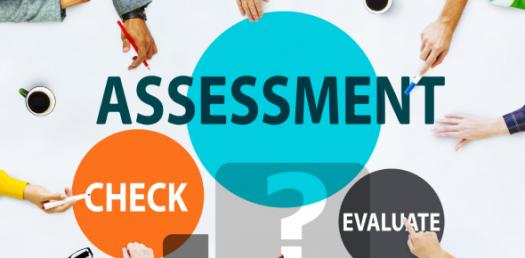# Volume & Surface Area Assessment Test

10 Questions | Total Attempts: 186SettingsThe volume and surface area of a certain 3D object help us measure the size and shape of the object. Take our assessment test to assess your knowledge of these mathematical measurements.

• 1.
What is the area of a circle?
• A.

• B.

Pi

• C.

• D.

• 2.
What is the volume of a rectangle?
• A.

• B.

Length × height

• C.

Width × length

• D.

• 3.
An example of a three dimensional shape is a...
• A.

Square

• B.

Line

• C.

Circle

• D.

Cube

• 4.
The quantity of three-dimensional space enclosed by a closed surface is called...
• A.

Plane

• B.

Point

• C.

Volume

• D.

Space

• 5.
What is the volume of a cylinder?
• A.

Area × height

• B.

Pi × height × weight

• C.

• D.

Length × height

• 6.
Area can be measured in which of the following?
• A.

Metre square

• B.

Cubic metre

• C.

Cm

• D.

Litres

• 7.
The extent of a two-dimensional figure or shape is called...
• A.

Plane

• B.

Surface

• C.

Area

• D.

Volume

• 8.
What is the area of a rectangle?
• A.

Length × volume

• B.

• C.

• D.

Pi × length

• 9.
What is the area of a triangle?
• A.

• B.

• C.

1/2 × Height × height

• D.

1/2 × Length × length

• 10.
What is the area of a sphere?
• A.

Length × height

• B.Back to top